Name:    Chapter 3 Online Practice

Multiple Choice
Identify the letter of the choice that best completes the statement or answers the question.

1.

Ammonia is prepared by reacting nitrogen and hydrogen gases at high temperature according to the unbalanced chemical equation below.

__ N2(g) + __ H2(g) ® __ NH3(g)

What are the respective coefficients when the equation is balanced with the smallest whole numbers?
 a. 1, 1, 1 b. 1, 3, 1 c. 1, 3, 2 d. 2, 1, 2 e. 2, 3, 2

2.

The reaction of calcium with elemental chlorine yields calcium chloride. Write a balanced chemical equation for this reaction.
 a. Ca(s) + Cl2(g) ® CaCl(s) + Cl(g) b. Ca(s) + Cl2(g) ® CaCl2(s) c. Ca(s) + 2 Cl2(g) ® CaCl4(s) d. 2 Ca(s) + Cl2(g) ® 2 CaCl(s) e. 4 Ca(s) + Cl2(g) ® 2 Ca2Cl(s)

3.

The balanced equation for the complete combustion of benzene, C6H6, is
 a. C6H6(l) ® 6 C(s) + 3 H2(g) b. C6H6(l) + 12 H2O(l) ® 6 CO2(g) + 15 H2(g) c. C6H6(l) + O2(g) ® CO2(g) + H2O(g) d. 2 C6H6(l) + 9 O2(g) ® 12 CO (g) + 6 H2O(g) e. 2 C6H6(l) + 15 O2(g) ® 12 CO2(g) + 6 H2O(g)

4.

Aluminum reacts with oxygen to produce aluminum oxide.

4 Al(s) + 3 O2(g) ® 2 Al2O3(s)

If 5.0 moles of Al react with excess O2, how many moles of Al2O3 can be formed?
 a. 1.0 mol b. 2.0 mol c. 2.5 mol d. 5.0 mol e. 10.0 mol

5.

Calcium reacts with fluorine gas to produce calcium fluoride. How many moles of Ca will react with 1.0 moles of F2?
 a. 0.50 mol b. 1.0 mol c. 1.5 mol d. 2.0 mol e. 4.0 mol

6.

Copper reacts with nitric acid to produce copper(II) nitrate, nitrogen dioxide gas, and water.

Cu(s) + 4 HNO3(aq) ® Cu(NO3)2(aq) + 2 NO2(g) + 2 H2O(l)

If you have 0.500 moles of Cu,
 a. you need at least 0.125 moles of HNO3 to produce 0.500 moles of Cu(NO3)2. b. you need at least 0.250 moles of HNO3 to produce 0.500 moles of Cu(NO3)2. c. you need at least 2.00 moles of HNO3 to produce 0.500 moles of Cu(NO3)2. d. you need at least 2.00 moles of HNO3 to produce 1.00 moles of Cu(NO3)2. e. you need at least 2.00 moles of HNO3 to produce 2.00 moles of Cu(NO3)2.

7.

Nitroglycerine decomposes violently according to the unbalanced chemical equation below. How many total moles of gases are produced from the decomposition of 1.00 mol C3H5(NO3)3?

C3H5(NO3)3(l) ® CO2(g) + N2(g) + H2O(g) + O2(g)
 a. 4.00 mol b. 6.50 mol c. 7.25 mol d. 16.5 mol e. 29.0 mol

8.

Dinitrogen trioxide, a blue solid, dissociates to form nitrogen monoxide and nitrogen dioxide gases. What mass of nitrogen dioxide is formed from the decomposition of 13.1 g of N2O3?
 a. 5.17 g b. 7.93 g c. 6.55 g d. 12.8 g e. 21.6 g

9.

What mass of carbon dioxide is produced by the complete combustion of 5.50 grams of hexane, C6H14?
 a. 0.468 g b. 2.81 g c. 3.06 g d. 16.9 g e. 33.7 g

10.

What is a correct method for determining how many grams of oxygen react with 1.00 gram of pentane?

C5H12(g) + 8 O2(g) ® 5 CO2(g) + 6 H2O(g)
 a. 1.00 g C5H12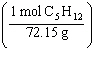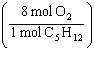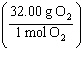= b. 1.00 g C5H12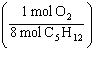= c. 1.00 g C5H12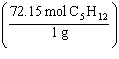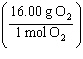= d. 1.00 g C5H12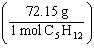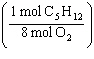= e. 1.00 g C5H12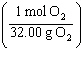=

11.

If 0.250 moles of bromine and 0.600 moles of ammonia react according to the equation below, what is the maximum amount of ammonium bromide (in moles) produced?

3 Br2(l) + 8 NH3(g) ® 6 NH4Br(s) + N2(g)
 a. 0.250 mol b. 0.450 mol c. 0.500 mol d. 0.600 mol e. 0.800 mol

12.

Sulfur trioxide is made from the reaction of sulfur dioxide and oxygen. How many grams of SO3 can be made from the reaction of 3.00 g SO2 with 2.02 g O2?

2 SO2(g) + O2(g) ® 2 SO3(g)
 a. 2.53 g b. 3.75 g c. 5.02 g d. 5.05 g e. 10.1 g

13.

The reaction of 10.0 g H2(g) with 10.0 g O2(g) yields 8.43 g H2O(g). What is the percent yield of this reaction?
 a. 9.43% b. 27.3% c. 42.2% d. 66.8% e. 74.9%

14.

Aspirin is produced by the reaction of salicylic acid (M = 138.1 g/mol) and acetic anhydride (M = 102.1 g/mol).

C7H6O3(s) + C4H6O3(l) ® C9H8O4(s) + C2H4O2(l)

If 2.04 g of C9H8O4 (M = 180.2 g/mol) is produced from the reaction of 3.00 g C7H6O3 and 5.40 g C4H6O3, what is the percent yield?
 a. 14.2% b. 21.4% c. 24.3% d. 52.1% e. 68.0%

15.

Under certain conditions the formation of ammonia from nitrogen and hydrogen has a 7.82% yield. Under these conditions, how many grams of NH3 will be produced from the reaction 25.0 g N2 with 2.00 g H2?

N2(g) + 3 H2(g) ® 2 NH3(g)
 a. 0.881 g b. 2.37 g c. 3.12 g d. 11.3 g e. 30.4 g

16.

A mass of 2.052 g of a metal carbonate, MCO3, is heated to give the metal oxide and 0.4576 g CO2.

MCO3(s) ® MO(s) + CO2(g)

What is the identity of the metal?
 a. Cu b. Mg c. Ca d. Ba e. Co

17.

A mixture of MgCO3 and MgCO3·3H2O has a mass of 3.057 g. After heating to drive off all the water the mass is 2.790 g. What is the mass percent of MgCO3·3H2O in the mixture?
 a. 22.4% b. 43.2% c. 67.0% d. 77.6% e. 91.3%

18.

A 4.236 g sample of a hydrocarbon is combusted to give 3.810 g of H2O and 13.96 g of CO2. What is the empirical formula of the compound?
 a. C2H3 b. C3H2 c. C3H4 d. C4H3 e. C5H12

19.

Cyclohexene, a hydrocarbon, has a molar mass of 82.1 g/mole. If the combustion of 0.8300 g cyclohexene produces 0.9102 g H2O and 2.668 g CO2, what is the molecular formula of this compound?
 a. C3H5 b. C5H22 c. C6H10 d. C6H14 e. C7H10